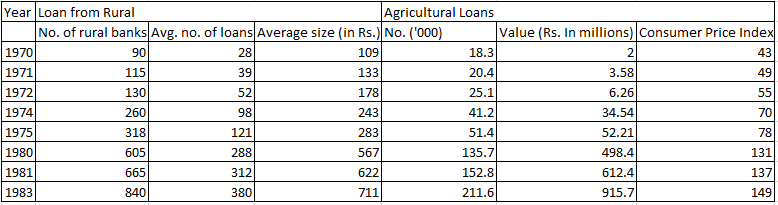### CAT 1997 Question Paper Question 24

Instructions

The table given below gives the annual details of loans from rural banks and agricultural loans over the years 1970 to 1983. Using this data answer the questions that follow.Question 24

# From the given data, the number of rural loans up to 1980 formed approximately what percentage of those in 1983?

Solution

Number of loans in 1970 = 90*28 = 2520

Number of loans in 1971 = 115*39 = 4485

Number of loans in 1972 = 130*52 = 6760

Number of loans in 1974 = 260*98 = 25480

Number of loans in 1975 = 318*121 = 38478

Number of loans in 1980 = 605*288 = 174240

Sum of these loans = 251963

Number of loans in 1983 = 840*380 = 319200

Ratio = $$\frac{251963}{319200}$$ = 80% approximately.